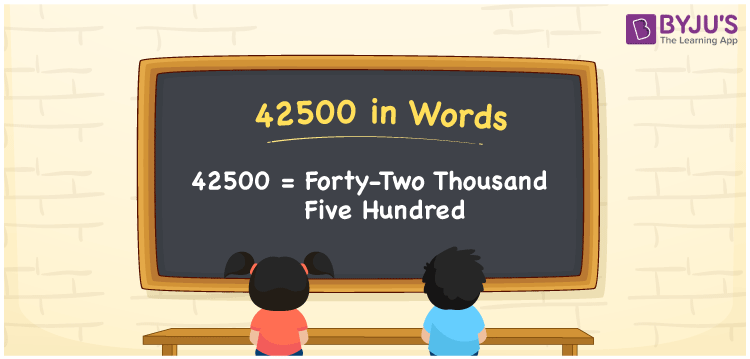# 42500 in Words

We can write 42500 in words as Forty-two thousand Five hundred. Suppose you have seen a refrigerator’s price tag, which is Rs. 42500, you may read it as Forty-two thousand five hundred rupees. In this article, you will learn how to write the cardinal number 42500 in words in an easily understandable method.

 42500 in words Forty-two thousand Five hundred Forty-two thousand Five hundred in Numbers 42500

## 42500 in English words

Generally, we can write numbers in words using the English alphabet. So, we can read 42500 in English as “Forty-two thousand Five hundred”.## How to Write 42500 in Words?

As we can see 42500 contains five digits, so we need to make a place value chart with 5 columns as shown below.

 Ten thousands Thousands Hundreds Tens Ones 4 2 5 0 0

From the above chart, we can write the digits according to their place values as:

4 × Ten thousand + 2 × Thousand + 5 × Hundred + 0 × Ten + 0 × One

= 4 × 10000 + 2 × 1000 + 5 × 100 + 0 × 10 + 0 × 1

= 40000 + 2000 + 500

= Forty thousand + Two thousand + Five hundred

= Forty-two thousand Five hundred

Thus, 42500 in words = Forty-two thousand Five hundred.

### Facts About the Number 42500

42500 is a natural number that is the successor of 42499 and the predecessor of 42501.

42500 in words – Forty-two thousand Five hundred

Is 42500 an odd number? – No

Is 42500 an even number? – Yes

Is 42500 a perfect square number? – No

Is 42500 a perfect cube number? – No

Is 42500 a prime number? – No

Is 42500 a composite number? – Yes

## Frequently Asked Questions on 42500 in Words

Q1

### How do you write 42500 in English words?

In English words, 42500 can be written as Forty-two thousand Five hundred.
Q2

### How do you mention 42500 in words on a cheque?

On a cheque, we generally write 42500 in words as Forty-two thousand Five hundred rupees only.
Q3

### What is the value of 30000 + 12500 in words?

30000 + 12500 = 42500 The value 30000 + 12500, i.e. 42500 in words is Forty-two thousand Five hundred.